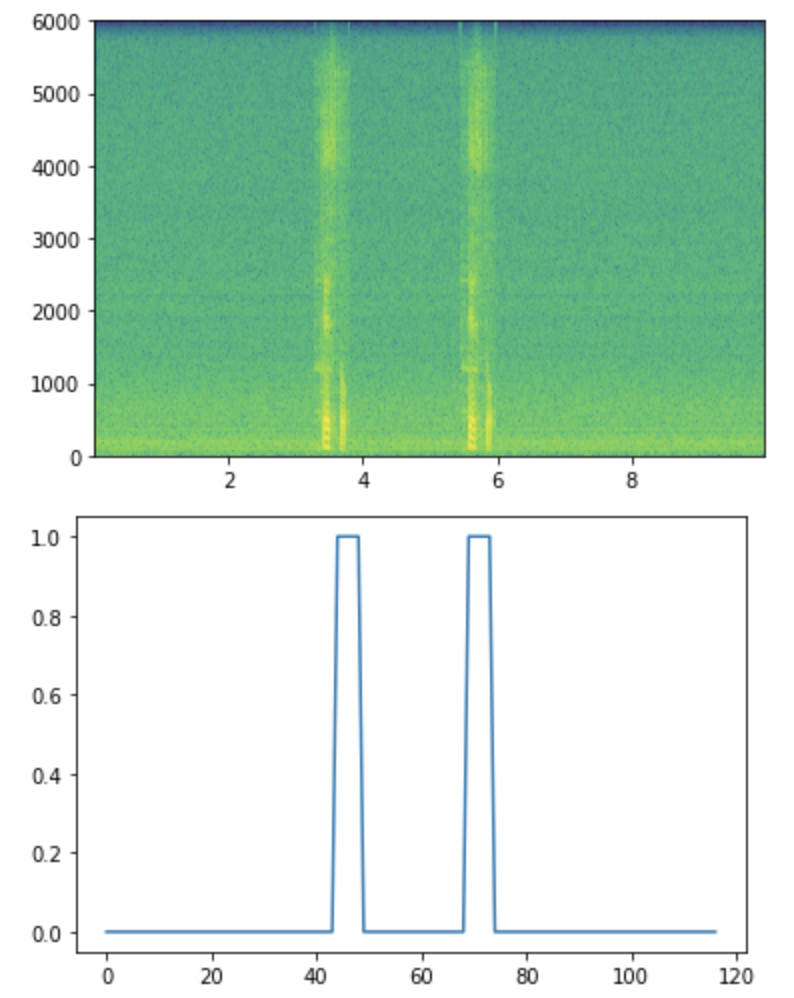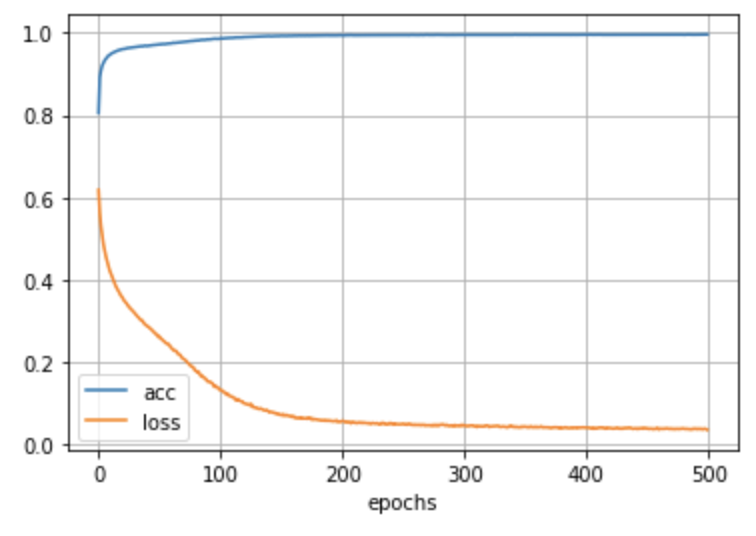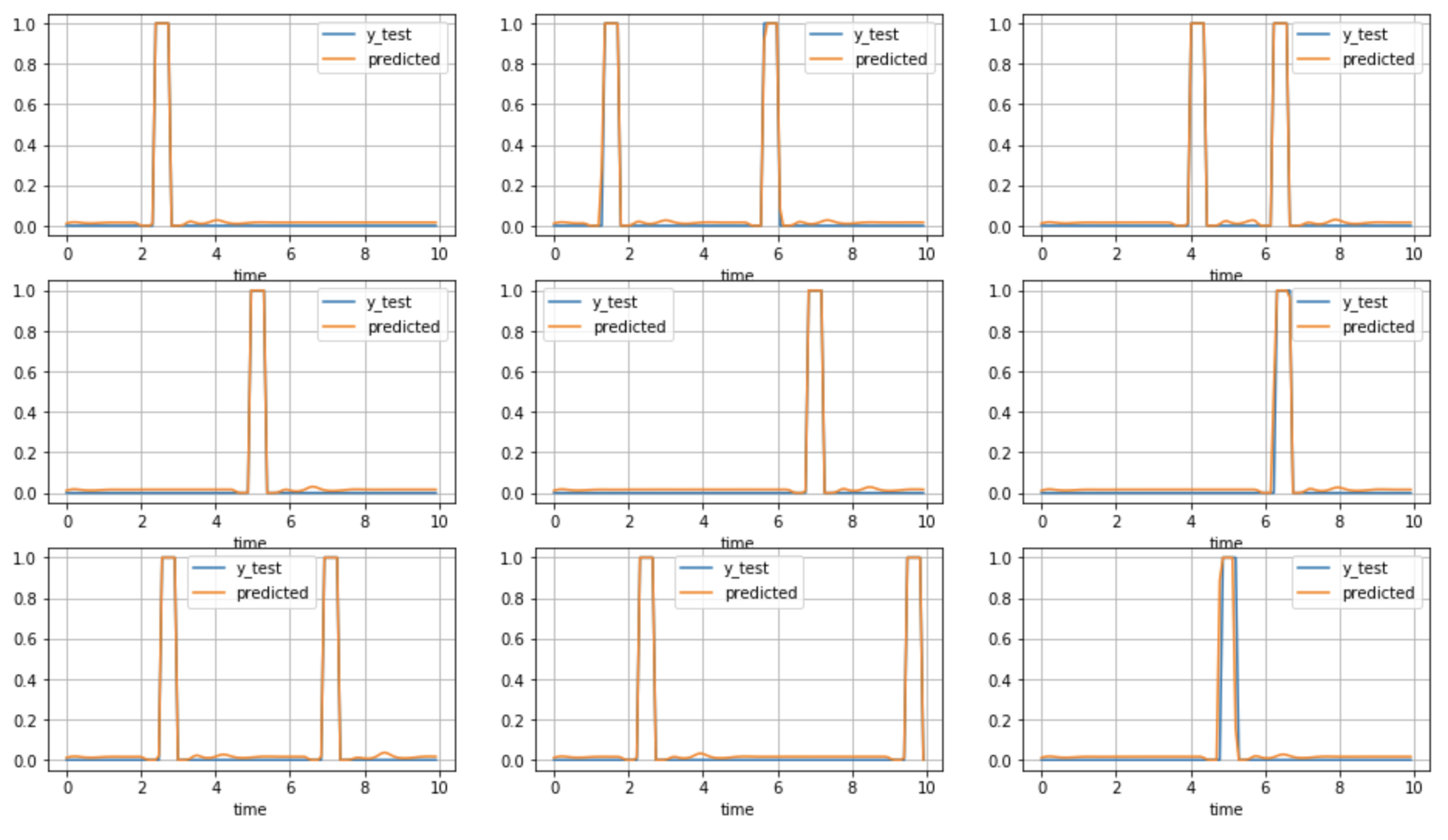# What went

happy New Year. This is a hobby study record I went to during my New Year's homecoming.

Normally, when using the voice assistant, I think it's OK to call with a wake word such as Google. When I received Deep Learning Specialization (Coursera) by Dr. Andrew Ng There was an issue with implementing a model that detects wakeward utterances.

This article is a review of the above course, It is a record that generated learning data based on the recorded data of one's voice and trained it with the implemented model. There is little data and the results are simple, but eventually I would like to increase the data and improve the model to experiment.

Deep Learning Specialization is voluminous but easy to understand. I recommend it because I was able to understand it even as a beginner.

# Execution environment

• Google Colaboratory --Runtime type: Python 3 --Hardware Accelerator: GPU
• Tensorflow ver 1.15.0

# Creating training data

## material

--Background noise.wav 2 types --Voice recording .wav --Two types of recordings of your own voice saying'TEST'(ground voice, falsetto)

## Contents

--Defined a function to generate a large amount of learning data from a small amount of material --Randomly change the volume of noise, the location where voice is combined, and the number (1 to 3) to secure variation

--What you get --Input data X: Spectrogram of synthetic sound source for 10s (number of time frames, number of frequency bins) --Correct label y: 0 or 1 flag ――The label about 40ms after the part where the voice was synthesized was set to 1. The rest is 0

## Generated data

--The above figure is a spectrogram that is input data (vertical: frequency [Hz] horizontal: time) ――The color of the corresponding part has changed due to the composition of the voice. ――This color shows the strength of the frequency component of the voice.--The figure below shows the generated correct label. --The label of the combined part is changed to 1. --Attempts to infer this from the input data

### Function for generation

A function defined for data generation

• make_train_sound(background, target, length, dumpwav=False) --Generate one synthetic sound source and one correct label
• make_train_pattern(pattern_num) --Tap make_train_sound multiple times to generate training data
``````BACKGROUND_DIR = '/tmp/background'
VOICE_DIR = '/tmp/voice'
RATE = 12000
Ty = 117
TRAIN_DATA_LENGTH = int(10*RATE)

def make_train_sound(background, target, length, dumpwav=False):
"""
arguments
background: background noise data
target: target sound data (will be added to background noise)
length: sample length
dumpwav: make wav data

output
X: spectrogram data  ( shape = (NFFT, frames))
y: flag data (shap)
"""
NFFT = 512
FLAG_DULATION = 5
TARGET_SYNTH_NUM = np.random.randint(1,high=3)

# initialize
train_sound = np.copy(background[:length])
gain = np.random.random()
train_sound *= gain
target_length = len(target)
y_size = Ty
y = [0 for i in range(y_size)]

# Synthesize
for num in range(TARGET_SYNTH_NUM):
# Decide where to add target into background noise
range_start = int(length*num/TARGET_SYNTH_NUM)
range_end = int(length*(num+1)/TARGET_SYNTH_NUM)
synth_start_sample = np.random.randint(range_start, high=( range_end - target_length - FLAG_DULATION*(NFFT) ))
train_sound[synth_start_sample:synth_start_sample + target_length] += np.copy(target)

# get Spectrogram
specgram, freqs, t, img = plt.specgram(train_sound,NFFT=NFFT, Fs=RATE, noverlap=int(NFFT/2), scale="dB")
X = specgram        # (freqs, time)

# Labeling
target_end_sec = (synth_start_sample+target_length)/RATE
train_sound_sec = length/RATE
flag_start_sample = int( ( target_end_sec / train_sound_sec ) * y_size)
flag_end_sample = flag_start_sample+FLAG_DULATION
if y_size <= flag_end_sample:
over_length = flag_end_sample-y_size
flag_end_sample -= over_length
duration = FLAG_DULATION - over_length
else:
duration = FLAG_DULATION
y[flag_start_sample:flag_end_sample] = [1 for i in range(duration)]

if dumpwav:
scipy.io.wavfile.write("train.wav", RATE, train_sound)

y = np.array(y)
return (X, y)

def make_train_pattern(pattern_num):
"""
return list of training data
[(X_1, y_1), (X_2, y_2) ... ]

arguments
pattern_num: Number of patterns (X, y)

output:
train_pattern: X input_data, y labels
[(X_1, y_1), (X_2, y_2) ... ]
"""
bg_items = get_item_list(BACKGROUND_DIR)
voice_items = get_item_list(VOICE_DIR)

train_pattern = []
for i in range(pattern_num):
item_no = get_item_no(bg_items)

item_no = get_item_no(voice_items)
pattern = make_train_sound(bgdata, voicedata, TRAIN_DATA_LENGTH, dumpwav=False)

train_pattern.append(pattern)

return train_pattern
``````

--Using these, 1500 data were generated and separated for train, validation, and test. --Since 1500 or more have exceeded the RAM of Colab and stopped, so far --In order to handle the input data as time series data, the shape was changed (number of time frames, number of frequency bins).

``````#Data creation
train_patterns = make_train_pattern(1500)

#Input from the obtained tuple,Divide into correct labels
X = []
y = []
for t in train_patterns:
X.append(t.T)    # (Time, Freq)
y.append(t)
X = np.array(X)
y = np.array(y)[:,:,np.newaxis]

train_patterns = None

# training, validation,Divide for test
train_num = int(0.7*len(X))
val_num = int(0.2*len(X))
test_num = int(0.1*len(X))

X_train = X[:train_num]
y_train = y[:train_num]
X_validation = X[train_num:train_num+val_num]
y_validation = y[train_num:train_num+val_num]
X_test = X[train_num+val_num:]
y_test = y[train_num+val_num:]
train_data_shape = X_train.shape

``````

# Model for learning

--Defined a model with one layer of CNN and two layers of LSTM. --input_shape is set to the size of the training data (spectrogram)

``````_________________________________________________________________
Layer (type)                 Output Shape              Param #
=================================================================
input_6 (InputLayer)         (None, 467, 257)          0
_________________________________________________________________
conv1d_6 (Conv1D)            (None, 117, 196)          755776
_________________________________________________________________
batch_normalization_16 (Batc (None, 117, 196)          784
_________________________________________________________________
activation_6 (Activation)    (None, 117, 196)          0
_________________________________________________________________
dropout_16 (Dropout)         (None, 117, 196)          0
_________________________________________________________________
cu_dnnlstm_11 (CuDNNLSTM)    (None, 117, 128)          166912
_________________________________________________________________
batch_normalization_17 (Batc (None, 117, 128)          512
_________________________________________________________________
dropout_17 (Dropout)         (None, 117, 128)          0
_________________________________________________________________
cu_dnnlstm_12 (CuDNNLSTM)    (None, 117, 128)          132096
_________________________________________________________________
batch_normalization_18 (Batc (None, 117, 128)          512
_________________________________________________________________
dropout_18 (Dropout)         (None, 117, 128)          0
_________________________________________________________________
time_distributed_6 (TimeDist (None, 117, 1)            129
=================================================================
Total params: 1,056,721
Trainable params: 1,055,817
Non-trainable params: 904
_________________________________________________________________
``````

--It seems that you can apply the fully connected layer to the time series by using TimeDistributed. --The fully connected layer of the activation function sigmoid is set in the final layer so that the probability is output.

``````X = TimeDistributed(Dense(1, activation='sigmoid'))(X)
``````

--CuDNNL STM was used to prioritize speed ――I changed it because the progress was slow when I trained with normal LSTM. --CuDNNLSTM did not work on Colab when using Tensorflow ver2.0 series --Is it possible to use it by importing from tf.compat.v1.keras.layers? --This time, I tried it with ver 1.15.0 to save time.

# Learning results

――We proceeded with the learning under the following conditions

``````detector = model(train_data_shape)
optimizer = Adam(lr=0.0001, beta_1=0.9, beta_2=0.999, decay=0.01)
detector.compile(loss='binary_crossentropy', optimizer=optimizer, metrics=["accuracy"])
history = detector.fit(X_train, y_train, batch_size=10,
epochs=500, verbose=1, validation_data=(X_validation, y_validation))
``````

――Learning proceeded in about 3s per epoch. High speed instead of CuDNNLSTM effect

``````Epoch 1/500
1050/1050 [==============================] - 5s 5ms/step - loss: 0.6187 - acc: 0.8056 - val_loss: 14.2785 - val_acc: 0.0648
Epoch 2/500
1050/1050 [==============================] - 3s 3ms/step - loss: 0.5623 - acc: 0.8926 - val_loss: 14.1574 - val_acc: 0.0733
``````

――It is a transition of learning. It seemed that I was able to learn enough around 200 epoch――The correct answer rate was high even in the TEST data.

``````detector.evaluate(X_test, y_test)
150/150 [==============================] - 0s 873us/step
[0.018092377881209057, 0.9983475764592489]
``````

--It seemed that the prediction was almost accurate when compared with the correct label of the TEST data (blue: correct answer orange: prediction). ――It's too predictable and something is wrong# in conclusion

――I deepened my understanding of the flow from data generation to learning. ――It became a simple model that only detects voice, but it was a learning experience.

--The amount and variation of training data was insufficient ――Since there is no variation in utterance data, it seems that it will react to other words. --Maybe it's just detecting the part you're synthesizing

――In the future, I think it would be good if we could improve the model and data for learning, and use the results to create apps.

Thank you very much. Have a nice year this year as well.